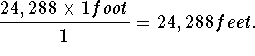# Units of ConversionLinear MeasureBasic to the understanding of unit conversion is the understanding equivalence, the understanding of the multiplicative identity of 1, and how the two are related.

Review the following table (Webster's New World Dictionary, Second Edition, Simon and Schuster, 1982) of Standard Units of Conversion to see if you can create fractions that have a value of 1. You can click to examples following each table.

 1 mil 0.001 inch 0.0254 millimeter 1 inch 1,000 mils 2.54 centimeters 12 inches 1 foot 0.3048 meter 3 feet 1 yard 0.9144 meter 5.5 yards or 16.5 feet 1 rod (or pole or perch) 5.029 meters 1 mile 5,280 feet 1.6094 kilometers 40 rods 1 furlong 201.168 meters 8 furlongs 1 mile 1.6094 kilometers 3 miles 1 league 4.83 kilometers 10 millimeters 1 centimeters 0.3937 inch 10 centimeters 1 decimeters 3.937 inches 10 decimeters 1 meters 39.37 inches or 3.20808 feet 10 meters 1 decameters 393.7 inches or 32.8083 feet 10 decameters 1 hectometers 328.083 feet 10 hectometers 1 kilometers 0.621 mile or 3,280 feet 10 kilometers 1 myriameter 6.21 miles

Example 1. Convert 4.6 miles to feet.

Solution.

• Write 4.6 miles as a fraction by dividing it by 1: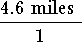.
• Multiply the fraction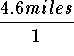by 1: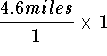• Note: We know that the fraction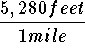is equivalent to 1 because the numerator and the denominator measure the same length.
• Convert the number 1 in the expressionto the equivalent fractionso that the expressioncan be written as• Rewrite 4.6 miles as 4.6 x 1 mile and 5280 feet as 5280 x 1 foot, and rewrite the two fractions as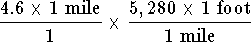• Multiply the two fractions: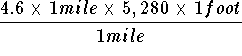• Rearrange the numerator: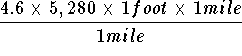• Write the above fraction as two fractions: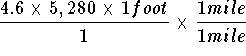• Replace the fraction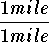by 1: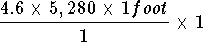• Simplify: